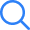# ML Aggarwal Solutions for Class 6 to 12 Maths Chapter Wise Free PDF

ML Aggarwal Maths Solutions for classes 6 to 12 eases the learning process of students. It aids in clearing the doubts, solving complex problems, covering maths syllabus and boosting the exam preparation process. It is one of the best reference textbooks solutions for the students studying in ICSE schools. Since, students are often in stress to complete their syllabus on time, due to it their learnings get affected. That is why here we have given the ML Aggarwal Solutions for ICSE classes 6 to 7 students.

These are developed as per the ICSE guidelines and it covers all the topics as prescribed by the board.

###### Select Language

In order to score good marks you are required to do practice as much as you can. It is one of the easiest ways to be well versed with all the questions available in the Maths Textbook and practice all of them. The solutions are available here in the organised way, firstly chapter wise and then exercise wise are given. Furthermore, keeping in mind the comprehending capability of a student’s age group, each and every single problem has been simplified.

Referring to it, the learners will improve their problem solving skills along with the new methodologies to answer the problems.

## ML Aggarwal Class 12 Solutions for ICSE Chapter Wise

The chapter wise ML Aggarwal textbook Solutions enable the student to easily navigate to the problems present in each chapter of mathematics. These will also help them in grasping the complex concepts much faster and easier. It also helps in revising the topics and answering their questions.

In ICSE 12th Class there are many topics such as Matrices, Boolean Algebra, Conics, Inverse Trigonometric Functions, and more. Having a good grip on these allows the students to prepare for the competitive exams.

## Class 11 Solutions

Class 11 ML Aggarwal Textbook contains various maths topics such as Sets, Relations and Functions, Trigonometric Functions, etc. All of these are extremely important for the XIth standard students who opt for Science stream. Therefore, the solutions prepared by our subject matter are given here. It will develop rational thinking and logical approach in students along with better understanding of concepts and its applications.

Those who refer to these, will be able to solve various questions including MCQs (Multiple Choice Questions), Short Type Questions, and Long Type of Questions too.

## ICSE Class 10 Maths Solutions

ICSE Class 10 Maths Solutions is a great study material for the board students. They can use it to start their board exam preparation and become able to score upto full marks. Solutions provide hand holding support to the learners, that is why it is suggested here to practice the maths questions thoroughly with the help of ML Aggarwal Solutions. The step wise answers to all the questions are provided here for 10th standard.

These are designed only for the class 10th students that allows them to practice more in less time.

### Advantages of using ML Aggarwal Maths Textbook Solutions are:

1. It provides well organised answers to each chapter and each question.
2. It helps in completing the full mathematics syllabus.
3. Improves problem solving skills. And more.

## FAQ. on ML Aggarwal Solutions for Class 6 to 12

Q:- Where will I find ML Aggarwal Class Wise Maths solutions?

A:- ML Aggarwal Class Wise Maths solutions are supposed to be solved by the students. However, in case if any stuck at some problems then they can find the ML Aggarwal Solutions for classes 6 to 12 on Selfstudys website.

Q:- Why to Use ML Aggarwal Solutions?

A:- ML Aggarwal Solutions are undoubtedly one of the best study resources to comprehend the complex topics of mathematics. Such problems are explained very precisely in step wise methods. Referring to enhance the students' understanding in the subjects. Furthermore, it helps in board exam preparation.×
Open Now